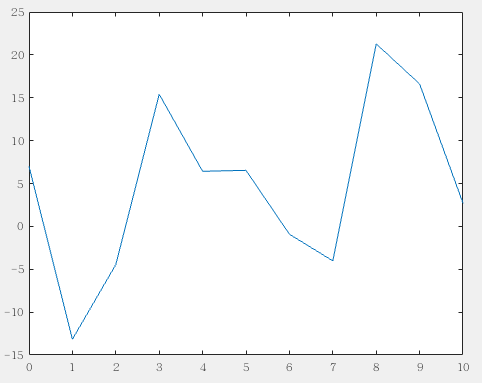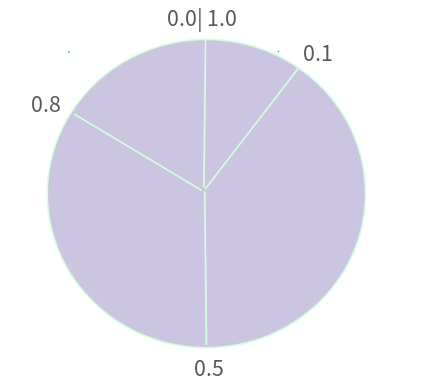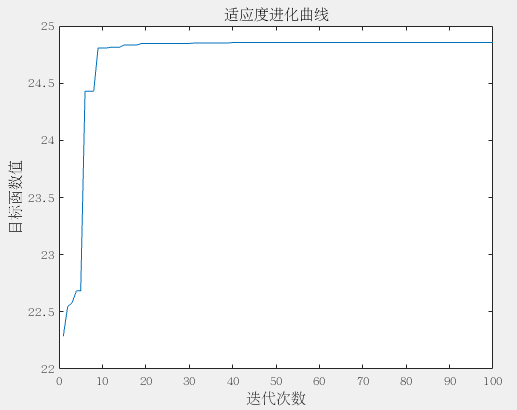# 遗传算法入门（中）实例，求解一元函数最值（MATLAB版）

6 篇文章 17 订阅

（上篇）遗传算法入门（上）代码中的进化学说与遗传学说
（下篇）遗传算法入门（下）C++求解TSP问题

# 目标函数分析

max:F(x)=x+10sin(5x)+7cos(4x),　x[0,10] m a x : F ( x ) = x + 10 s i n ( 5 x ) + 7 c o s ( 4 x ) , 　 x ∈ [ 0 , 10 ] $max :F(x) = x + 10sin(5x) + 7cos(4x) , x\in[0 , 10]$# 生成初始种群：编码VS解码

f=randi([0,1],NP,L);  %随机获得初始种群矩阵

for i=1:NP
U =f(i,:);
m=0;
for j=1:L
m=U(j)*2^(j-1)+m;
end
x(i)=Xx+m*(Xs-Xx)/(2^L-1);  %x(i)为[0,10]内的实数
end

# 关于适应度函数

​ 适应度函数是为了更好的编写代码，更好的参与选择、交叉和变异算子而在目标函数的基础上做的一系列改进，本文要求解的目标函数非常普通，而且本文求解的是最大值，即，个体的目标函数值越大，说明该个体越好，适应度就越好，所以就直接把目标函数当做适应度函数，本文提到的适应度函数就是目标函数。

​ 需要提一下的是，源码中对适应度值做了归一化的处理。大家可不用理会 。

# 选择策略

​ 在遗传算法中，选择策略用的最多的就是轮盘赌选择，也叫赌轮选择或者适应度比例选择法 。轮盘赌选择的原理并不难理解，我们这里给出一个简单的理解，详细的探讨将留在”深入遗传算法系列“中 。

​ 假设我们有4个个体个体：A 个体：B 个体：C 个体：D，其适应度、选择概率、累计概率如下表所示 ：

A100.10.1
B400.40.5
C300.30.8
D200.21​ 以上的思想就是轮盘赌的思想，其实还有另一个方式玩这个游戏，就是把骰子固定不动，我们转NP次轮盘，骰子指向哪个区域，就选中对应区域的个体。貌似这种方式只是变换了一下参考系而已。

​ 用代码实现上述的思想，也就会有两种方式，希望大家在看别的代码实现的时候能够搞清楚是哪两种方式。下面是MATLAB代码实现： （用的是轮盘转，骰子不动的思维方式）

sum_Fit = sum(Fit) ;
fitvalue = Fit ./sum_Fit ;   %选择概率，将Fit的每个元素除以sum_Fit
fitvalue = cumsum(fitvalue) ; %累积概率
ms = sort (rand(NP,1)) ;   %产生NP个0~1之间的随机数，并且升序排序
fiti =1;
newi=1;
while newi<=NP  %轮盘开始转，一共转NP次
if(ms(newi)<fitvalue(fiti))    %如果骰子落在轮盘的这个区域
nf(newi,:) = f(fiti , :) ; % 把个体给新的种群
newi = newi + 1;
else
fiti=fiti +1;
end
end

# 交叉与变异机制

for i = 1:2:NP   % 从1到NP ，每隔两个
p = rand ;
if p<Pc
q=randi([0,1],1,L);
for j =1:L
if q(j) == 1
temp=nf(i+1,j);
nf(i+1,j) = nf(i,j);
nf(i,j) = temp;
end
end
end
end

i = 1;
while i<=round(NP*Pc)   % 四舍五入
h=randi([1,NP],1,1) ; %随机选取一个需要变异的染色体
for j = 1:round(L*Pc)
g = randi([1,20],1,1);%随机选取需要变异的基因数
%g=rand;
nf(h,g) = ~ nf(h,g);
end
i=i+1;
end

# MATLAB绘图得出结果11-0910-1640万+
09-121万+
09-221816
03-20186
12-024333
06-05
05-254254
12-29
08-201056
05-22
12-094953
04-225万+
08-152773
11-19
07-10897点击重新获取扫码支付余额充值